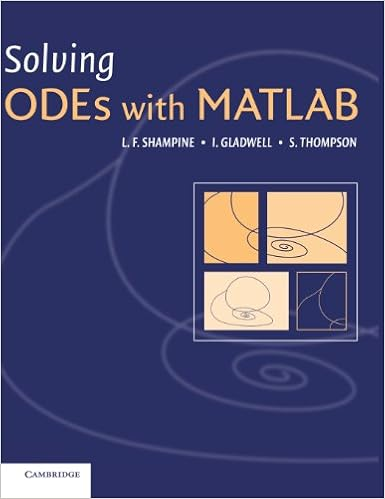## 耶鲁基础物理4.6连接体- 阅读剩余部分 -

## 优化变分迭代法解Emden-Lane边值问题一例

Emden-Lane边值问题：

\begin{equation} \begin{split} & u''(x)+\frac{\alpha}{x}u'(x)=f(x,u) \\ & u'(0)=0, au(1)+bu'(1)=c \end{split} \label{Emden-Lane} \end{equation}

- 阅读剩余部分 -

## 证明32.5=31.5- 阅读剩余部分 -

## 水立方## 用Matlab bvp4c 解带未知参数的常微分方程边值问题，怎么给出合适的初值？\begin{equation*} \begin{split} f'''-R[(f')^2-ff'']+RA=&0\\ R''+Rfh'+1=&0\\ \theta ''+P_ef\theta '=&0 \end{split} \end{equation*}

\begin{equation*} \begin{split} f(0)=f'(0)=&0\\ f(1)=f'(1)=&1\\ h(0)=h(1)=&0\\ \theta(0)=&0\\ \theta(1)=&1 \end{split} \end{equation*}

- 阅读剩余部分 -

## 文科教育为什么不能偏废？## 熵的勒让德变换《现代统计力学导论》练习1.6 构造熵的勒让德变换

- 阅读剩余部分 -

## 拍的音频演示

\begin{equation*} x_1=A\cos (\omega_1 t) \end{equation*}

\begin{equation*} x_2=A\cos (\omega_2 t) \end{equation*}

\begin{equation*} x=x_1+x_2=A\cos (\omega_1 t)+A\cos (\omega_2 t)=2A\cos\left(\frac{\omega_1-\omega_2 }{2} t\right)\cos\left(\frac{\omega_1+\omega_2 }{2}t \right) \end{equation*}